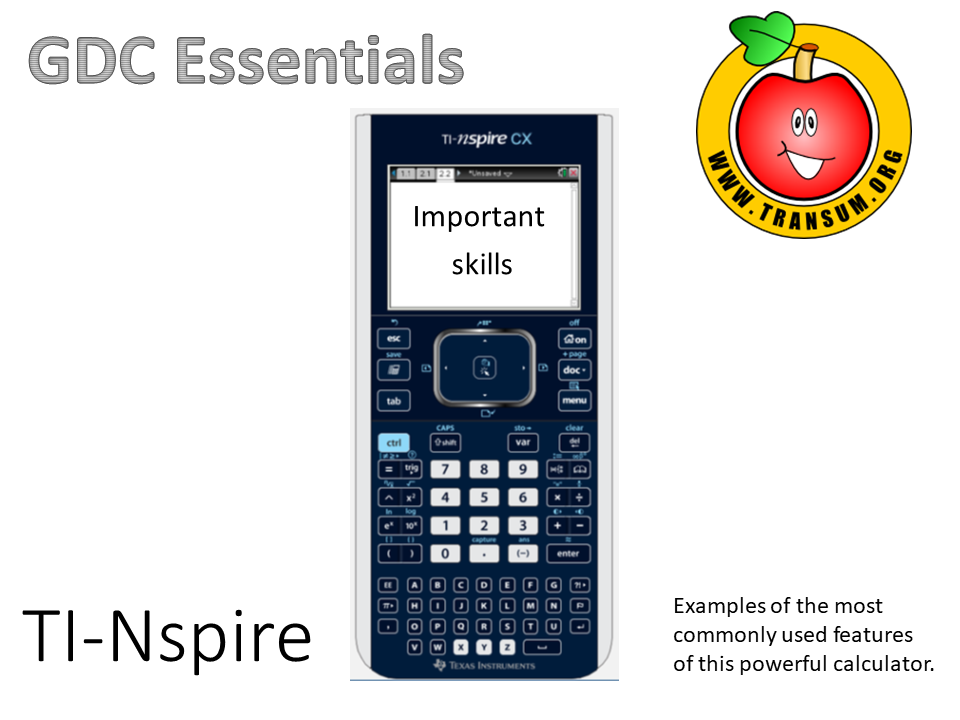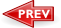Here is the URL for some more practice using your calculator.

Transum.org/go/?to=UsingCalc

# TI-Nspire CX Essential Skills (52 slides)

## Learn a skill a day for twenty days. There is a printable checklist to go with this presentation.Go directly to a particular skill:

Day 1 Solve simultaneous equations
Day 3 Standard form and variables
Day 4 Factors and multiples
Day 5 Time calculations
Day 6 Defining a function
Day 7 Generating a Sequence
Day 8 Calculating statistics from a list
Day 9 Calculating statistics from a frequency table
Day 10 Box plot from frequency table
Day 11 Plotting a graph and rescaling axes
Day 12 Finding a zero
Day 13 Solving simultaneous equations graphically
Day 14 Finding maximum and minimum points
Day 15 Horizontal asymptote and intervals
Day 16 Using nSolve to find a specific solution
Day 17 Scatter graphs and regression lines
Day 18 Spearman's rank correlation coefficient
Day 19 The normal distribution
Day 20 The binomial distribution
Day 21 The chi squared test for independence
Day 22 Finding the t-Test statistic
Day 23 Finance solver
Day 24 Finding the gradient of a graph (differentiation)
Day 25 Find the area bounded by a graph (integration)

Go directly to a particular slide:

This presentation as a PowerPoint file is available to teachers who have subscribed to Transum.

Here is the URL of this presentation linking directly to the slide you are currently viewing, slide number 1. Copy and paste this link into your scheme of work, revision notes or blog.

There are many more mathematical visual aids to project onto a whiteboard or screen in our Shine+Write area.